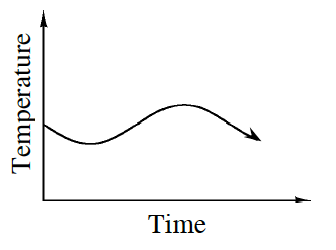### Home > CCA2 > Chapter Ch1 > Lesson 1.1.2 > Problem1-18

1-18.

Does the temperature outside depend on the time of day, or does the time of day depend on the temperature outside? This may seem like a silly question, but to sketch a graph that represents this relationship, you first need to decide which axis will represent which quantity. Homework Help ✎

1. When you graph an equation such as $y=3x-5$, which variable ($x$ or $y$) depends on the other? Which is not dependent? (That is, which is independent?) Explain.

The $x$ is independent and the $y$ is dependent because $y$ depends on $x$.

2. Which variable is dependent: temperature or time of day? Which variable is independent?

Reread the questions in the opening paragraph of the problem.

The time of day is independent and the temperature is dependent.

3. Sketch a graph (with appropriately named axes) that shows the relationship between temperature outside and time of day.

Each day starts at midnight.